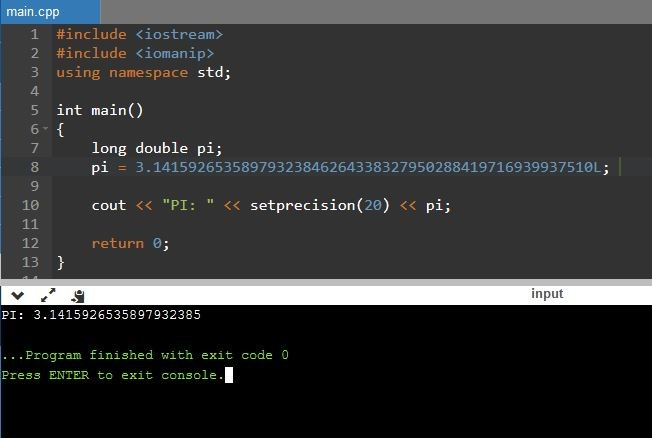# What is the Difference Between double and long double

The main difference between double and long double is that double is used to represent a double precision floating point while long precision is used to represent extended precision floating point value.

When writing programs, it is necessary to store data. Languages such as C++ use variables in the program. Variable is the name given to a location that stores data. Moreover, each variable has a data type that it can store a value. It denotes how much memory to allocate for a memory location. Furthermore, double and long double are two main data types. The amount of memory allocated by double and long double may vary depends on the system, but usually double allocates 8 bytes and long double allocates 12 bytes to data.

### Key Areas Covered

1. What is double
– Definition, Functionality
2. What is long double
– Definition, Functionality
3. Difference Between double and long double
– Comparison of Key Differences

### Key Terms

double, Floating Point, long double, Variable## What is double

The double is a data type that is used to store 64-bit double precision floating point value. Usually, it allocates 8 bytes of memory to the data.Figure 1: C++ program with double

In the above program, width and height are two double variables. The width variable stores 4.3 while height variable stores 2.5. Area is another variable of type double. The value calculated using width and height is assigned to the area variable. Finally, the cout statement displays the area on the console.

## What is long double

The long double is used to represent extended precision floating point value. Usually, it allocates 12 bytes to the data.Figure 2: C++ program with long double

According to the above program, pi is a variable of type long double. The programmer can declare the value with ‘L’ to denote the long double. The cout statement helps to display the PI value on the console after setting the precision using the setprecision method.

## Difference Between double and long double

### Definition

The double is a data type which is a double precision 64 bit IEEE 745 floating point while the long double is a floating point data type that is more precise than double precision. Thus, this explains the main difference between double and long double.

### Memory requirement

Another difference between double and long double is that double allocates 8 bytes to store data while long double allocates 12 bytes to store data.

### Precision

Moreover, long double provides more precision than double. Hence, this is also a difference between double and long double.

### Conclusion

The double and long double are two data types used in programming languages such as C++. The main difference between double and long double is that double is used to represent a double precision floating point while long precision is used to represent extended precision floating point value. In brief, long double provides more precision than double.

##### References:

1.“C Data Types.” GeeksforGeeks, 15 Nov. 2018, Available here.
2.“Long Double.” Wikipedia, Wikimedia Foundation, 3 Apr. 2019, Available here.